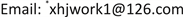1. 引言

2. 研究方法

ρ i n d ( r , ω ) = ∫ Π ( r , r ′ , ω ) V ( r ′ , ω ) d r ′ (1)

∫ Π ( r , r ′ , ω ) = 2 e 2 ∑ n m f ( E n ) − f ( E m ) E n − E m − ω − i γ ψ n ∗ ( r ) ψ m ( r ) ψ m ∗ ( r ′ ) ψ n ( r ′ ) (2)

V i n d ( r , ω ) = 1 4 π ε 0 ∫ ρ i n d ( r ′ , ω ) | r − r ′ | d r ′ (3)

V i n d ( r , ω ) = 2 e 2 ∑ n m f ( E n ) − f ( E m ) E n − E m − ω − i γ × 1 4 π ε 0 ∫ ψ n ∗ ( r ′ ) ψ m ( r ′ ) | r − r ′ | d r ′ [ V n m e x t ( ω ) + V n m i n d ( ω ) ] (4)

V n m ( ω ) = ∫ ψ n ∗ ( r ) V ( r , ω ) ψ m ( r ) (5)

V n ′ m ′ i n d ( ω ) = 2 e 2 ∑ n m f ( E n ) − f ( E m ) E n − E m − ω − i γ × 1 4 π ε 0 ∫ ψ n ∗ ( r ′ ) ψ m ( r ′ ) ψ n ′ ∗ ( r ) ψ m ′ ( r ) | r − r ′ | [ V n m e x t ( ω ) + V n m i n d ( ω ) ] d r ′ d r (6)

M n ′ m ′ , n m ( ω ) = 2 e 2 4 π ε 0 ∑ n m f ( E n ) − f ( E m ) E n − E m − ω − i γ ∫ ψ n ′ ∗ ( r ) ψ m ′ ( r ) ψ n ∗ ( r ′ ) ψ m ( r ′ ) | r − r ′ | d r ′ d r (7)

∑ n m [ δ n ′ m ′ , n m − M n ′ m ′ , n m ( ω ) ] V n m i n d ( ω ) = ∑ n m M n ′ m ′ , n m ( ω ) V n m e x t ( ω ) (8)

A ( ω ) = − 1 2 ω Im { ∫ ρ i n d ( r , ω ) [ V e x t ( r , ω ) ] ∗ } (9)

ψ n k ( r , θ ) = 1 π R 0 2 J n ( x n k R 0 2 r ) J n − 1 ( x n k ) e ± i n θ (10)

E n k = ℏ 2 x n k 2 2 m e R 0 2 (11)

ψ n k ( r , θ ) = C R n ( N k r ) e ± i n θ (12)

ρ i n d ( r , θ , ω ) = ∑ m ρ m i n d ( r , ω ) e i m θ (13)

V i n d ( r , θ , ω ) = ∑ m V m i n d ( r , ω ) e i m θ (14)

ρ m i n d ( r , ω ) = 4 π e 2 [ ∑ n k , k ′ ( 1 E n k − E ( n + m ) k ′ − ω − i γ + 1 E n k − E ( n + m ) k ′ + ω + i γ ) ] × C 2 R n ( N k r ) R n + m ( N k ′ r ) [ V n k k ′ e x t ( ω ) + V n k k ′ i n d ( ω ) ] (15)

V m i n d ( r , ω ) = ∫ r ′ ρ m i n d ( r ′ , ω ) e i m ( θ − θ ′ ) 4 π ε 0 r 2 + r ′ 2 − 2 r r ′ cos ( θ − θ ′ ) d r ′ d ( θ − θ ′ ) (16)

V n k k ′ ( ω ) = C 2 ∫ r ′ R n + m ( N k ′ r ′ ) R n ( N k r ′ ) V m ( r ′ , ω ) d r ′ (17)

∑ n k k ′ [ δ a b b ′ − M a b b ′ , n k k ′ ( ω ) ] V n k k ′ i n d ( ω ) = ∑ n k k ′ M a b b ′ , n k k ′ ( ω ) V n k k ′ e x t ( ω ) (18)

3. 结果与分析

4. 结论